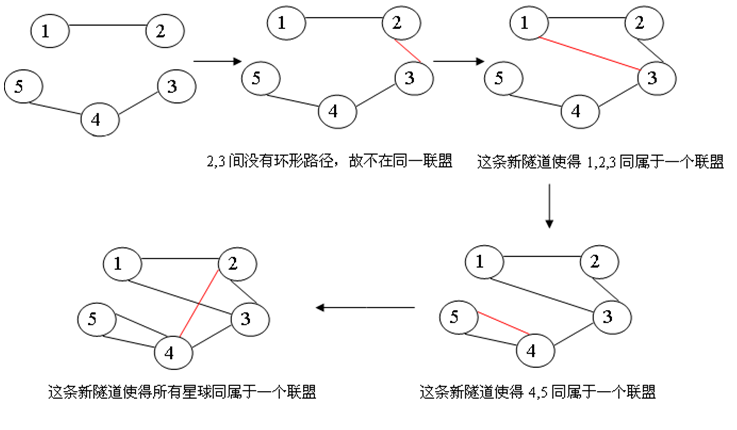# bzoj 4998 星球联盟 题解【LCT】【tarjan】

## Input

$1≤N,M,P≤200000$

## Sample Input

5 3 4
1 2
4 3
4 5
2 3
1 3
4 5
2 4


## Sample Output

No
3
2
5


## HINT## 题解：

$fa[u]=0$一定是最重要的！！！

## Code：

#include<cstdio>
#include<cstring>
#define ls Find(ch[k])
#define rs Find(ch[k])
#define which(k) (ch[Find(fa[k])]==k)
#define isroot(k) (Find(ch[Find(fa[k])])!=k&&Find(ch[Find(fa[k])])!=k)
int ch,fa,num;
int lazy,sz,s;
int Find(int k)
{
return (s[k]==k)?k:(s[k]=Find(s[k]));
}
void Union(int x,int y)
{
s[Find(y)]=Find(x);
}
void pushdown(int k)
{
if(lazy[k])
{
lazy[k]=0;
lazy[ls]^=1;
lazy[rs]^=1;
int tmp=ls;
ch[k]=rs;
ch[k]=tmp;
}
}
void maintain(int k)
{
sz[k]=sz[ls]+num[k]+sz[rs];
}
void Rotate(int k)
{
int y=Find(fa[k]);
if(!isroot(y))
ch[which(y)][Find(fa[y])]=k;
bool d=which(k);
fa[k]=Find(fa[y]);
fa[y]=k;
ch[d][y]=ch[!d][k];
fa[ch[d][y]]=y;
ch[!d][k]=y;
maintain(y);
maintain(k);
}
int stk,tp=0;
void splay(int k)
{
while(!isroot(k))
{
stk[++tp]=k;
k=Find(fa[k]);
}
stk[++tp]=k;
while(tp)
pushdown(stk[tp--]);
k=stk;
while(!isroot(k))
{
int y=Find(fa[k]);
if(!isroot(y))
Rotate(which(k)^which(y)?k:y);
Rotate(k);
}
}
void access(int k)
{
for(int x=k,y=0;x;y=x,x=Find(fa[x]))
{
splay(x);
ch[x]=y;
maintain(x);
}
}
void makeroot(int k)
{
access(k);
splay(k);
lazy[k]^=1;
}
int getroot(int k)
{
access(k);
splay(k);
while(ls)
k=ls;
return k;
}
void split(int x,int y)
{
makeroot(x);
access(y);
splay(y);
}
{
makeroot(x);
fa[x]=y;
}
int dfs(int k,int x)
{
int ans=num[k];
Union(x,k);
if(ls)
ans+=dfs(ls,x);
if(rs)
ans+=dfs(rs,x);
return ans;
}
{
x=Find(x),y=Find(y);
int ans=0;
makeroot(x);
if(getroot(y)!=x)
else
{
split(x,y);
ans=dfs(y,x);
num[x]=ans;
maintain(x);
fa[x]=0;
}
return ans;
}
int main()
{
int n,m,p,u,v;
scanf("%d%d%d",&n,&m,&p);
for(int i=1;i<=n;++i)
s[i]=i,num[i]=sz[i]=1;
for(int i=1;i<=m;++i)
{
scanf("%d%d",&u,&v);
}
for(int i=1;i<=p;++i)
{
scanf("%d%d",&u,&v);
if(tmp)
printf("%d\n",tmp);
else
puts("No");
}
return 0;
}


### 说点什么Subscribe

/* */